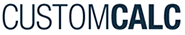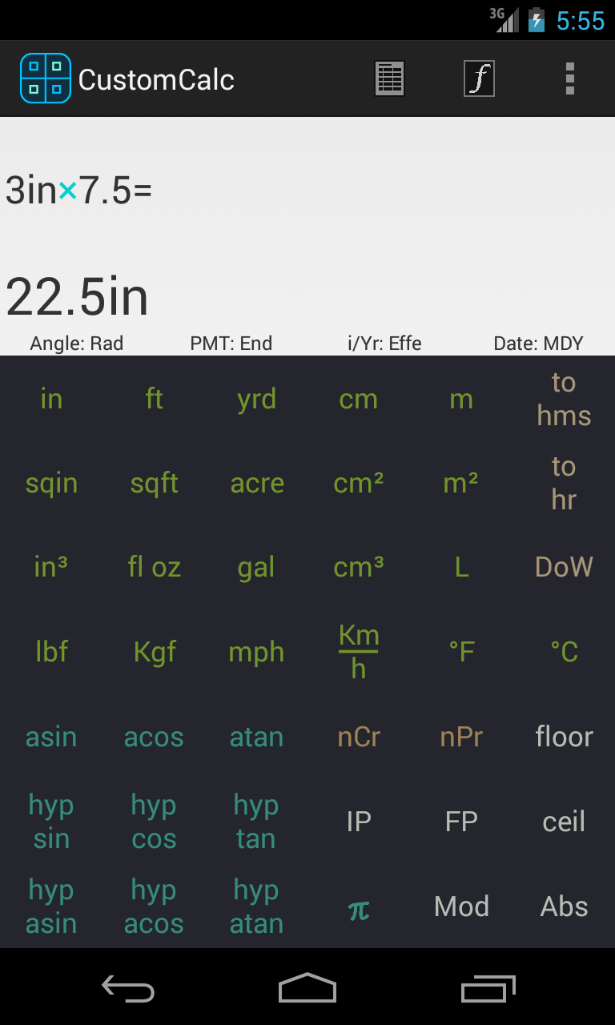﻿ CustomCalc - Android Custom Calculator••# CustomCalc Unit Conversion

CustomCalc offers 10 dimensional groups listed above to convert from any unit to another.

Any of these Unit Conversion Function can be assigned to any place in the keyboard (as any other in CustomCalc) so you can execute it in just one click (or touch), avoiding the need to browse for it.

Also in CustomCalc, all functions are available though the menu so you don't need to assign a function to the keyboard to execute it only once.

Dimensional units group

• Inches (in)
• Feet (ft)
• Yards (yrd)
• Miles (mi)
• Nautical miles (ntm)
• Angstrom (ang)
• Microns (mic)
• Millimeters (mm)
• Centimeters (cm)
• Meters (m)
• Kilometers (Km)
• Square inches (sqin)
• Square feet (sqft)
• Square yards (yrd²)
• Square miles (mi²)
• Acres (acre)
• Square nanometers (nm²)
• Square millimeters (mm²)
• Square centimeters (cm²)
• Square meters (m²)
• Hectars (Ha)
• Square kilometers (Km²)
• Cubic inches (in³)
• Cubic feet (ft³)
• Cubic yards (yrd³)
• Fluid ounces (fl oz)
• Pints (pint)
• Quarters (qrt)
• Gallon (gal)
• Gallon UK (gal UK)
• Cubic centimeters (cm³ / cc)
• Liters (L)
• Cubic meters (m³)
• Hectoliters (HL)
• Teaspoon (tea)
• Tablespoon (tbl)
• Cups (cup)
• Fluid ounces UK (fl oz UK)
• Pints UK (pint UK)
• Quarters UK (qrt UK)
• Barrels (barrel)
• Acre feets (acr ft)
• Ounces (oz)
• Pounds (lb)
• Milligrams (mg)
• Grams (g)
• Kilograms (Kg)
• Tons (metric) (ton)
• Tons short (ton short)
• Tons long (ton long)
• Grains (gr)
• Drams (dr)
• Drams Troy (dr troy)
• Ounces Troy (oz troy)
• Pound Troy (lb troy)
• Stones (sto)
• Slugs (slu)
• Carats (metric) (crt)
• Electro Volts (mass) (eV)
• Kiloelectro Volts (KeV)
• Megaelectro Volts (MeV)
• Ounces force (ozf)
• Pounds force (lbf)
• Kips (kip)
• Poundals (pnd)
• Tons force short (tonf short)
• Dynes (dyne)
• Millinewtons (mN)
• Newtons (N)
• Kilonewtons (KN)
• Grams force (gf)
• Kilograms force (Kgf)
• Kilogram·meters per sec (Kg·m/s)
• Tons force (tonf)
• Joul per centimeters (J/cm)
• Joul per meters (J/m)
• Pounds per square inch (psi)
• Pounds per square feet (lbf/ft²)
• Inches of Hg (in Hg)
• Inches of Water (in H2O)
• Kips per square inch (kip/in²)
• Atmospheres (atm)
• Millibars (mbar)
• Bars (bar)
• Newtons per square millimeters (N/mm²)
• Pascals (Newtons per square meters) (Pa)
• Kilopascals (KPa)
• Megapascals (MPa)
• Grams force per square centimeter (gf/cm²)
• Kilograms force per square centimeter (Kgf/cm²)
• Dynes per square centimeter (dyne/cm²)
• Centimeters of Hg (cm Hg)
• Centimeters of Water (cm H2O)
• Torrs (torr)
• Fahrenheit (°F)
• Celsius (°C)
• Kelvin (°K)
• Rankine (°R)
• BTUs per second (BTU/s)
• BTUs per hour (BTU/h)
• Feet pounds per second (ft·lbf/s)
• Feet pounds per hour (ft·lbf/h)
• Horse power (hp)
• CVs (CV)
• Calories per second (cal/s)
• Milliwatts (mW)
• Watts (W)
• Kilowatts (KW)
• Megawatts (MW)
• Tons refrigeration (ton refrig)
• Inches per second (in/s)
• Feet per second (ft/s)
• Feet per minute (ft/min)
• Miles per minute (mi/min)
• Miles per hour (mph)
• Millimeters per second (mm/s)
• Centimeters per second (cm/s)
• Meter per second (m/s)
• Kilometers per second (Km/s)
• Kilometers per hour (Km/h)
• Knots (knot)
• Calories (cal)
• Kilocalories (Kcal)
• Millijoules (mJ)
• Joules (J)
• Kilojoules (KJ)
• Megajoules (MJ)
• Horse power hour (hp·h)
• Watts hour (W·h)
• Kilowatts hour (KW·h)
• British Thermal Unit (BTU)
• ElectroVolts (eV)
• Mega electroVolts (MeV)
• Feet poundforce (ft·lbf)